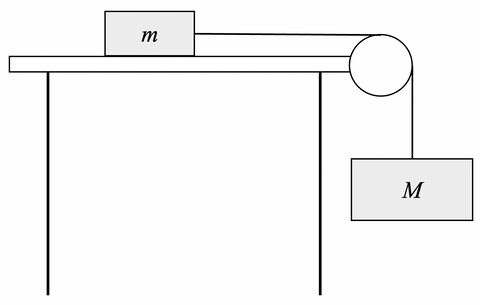# Systems Involving Multiple Masses

This topic is part of the HSC Physics course under the section Forces, Acceleration and Energy.

### HSC Physics Syllabus

• explore the concept of net force and equilibrium in one-dimensional and simple two-dimensional contexts using: (ACSPH050)

– vector addition by resolution into components
• solve problems or make quantitative predictions about resultant and component forces by applying the following relationships:

– F_{AB} = -F_{BA}

– F_x=Fcosθ, F_y=Fsinθ

### Treating Multiple Masses As A Single Mass

In some HSC Year 11 physics problems, it can be advantageous to consider a system of multiple masses as a single entity. This simplification often makes it easier to apply the principles of motion and conservation laws.

To treat multiple masses in a system as one mass, you should:

1. Combine the Masses: Add up the individual masses to get a total mass. This is the equivalent mass of the system.

2. Analyse the System: Apply Newton's laws or conservation laws to the entire system rather than to individual parts.

For example, if you are using Newton's second law, F = ma, the force (F) is the net external force acting on the system, the mass (m) is the total mass of the system, and the acceleration (a) is the common acceleration of the entire system.

3. Define Directions: In many cases, masses in a system may move in different directions or axes. Determine which direction will be positive and which will be negative before writing equations.

4. Consider Internal Forces: When analysing the system as a whole, internal forces (like the tension between connected masses) are not included in the force calculation. This is because they occur in equal and opposite pairs and therefore cancel out according to Newton's third law. Even if internal forces such as tension are included in the equation, they will cancel out algebraically.

By treating the system of multiple masses as a single mass, you can often simplify your analysis. However, it's important to remember that this method is not always applicable or helpful, especially if you need to find quantities specific to individual masses (like tension in a rope between two masses).

### Multi-mass System on a Frictionless Horizontal Surface

Consider two masses m and Mlies on a horizontal frictionless table surface tied to M via a rope and pulley system. M is hanging from the rope by the side of the table.The two masses must move in the same direction with equal acceleration in order to maintain the tension in the rope between them.

The acceleration is given by

$$a = \frac{F_{net}}{m_{total}}$$

The net force is sum of forces acting on m and M which can be identified by drawing a free body diagram.Let's define the downward direction of M as positive and upward direction as negative. This means the positive direction for m would be to the right, and the negative direction for M would be to the left.

$$a = \frac{Mg - T + T}{m + M}$$

$$a = \frac{Mg}{m + M}$$

### Multi-mass System on a Horizontal Surface with FrictionIn most cases, frictional force also acts on the mass on the horizontal surface, in the opposite direction to tension. Friction force should also be included in the free body diagram and equation.

$$a = \frac{Mg - T + T - f}{m + M}$$

Since friction is related to the normal force:

$$f = \mu N$$

For ﻿m﻿, its weight force balances the normal force because it is moving horizontally with no acceleration in the vertical axis. Therefore,

$$f = \mu mg$$

$$a = \frac{Mg - \mu mg}{m + M}$$

More examples are demonstrated in the video above.